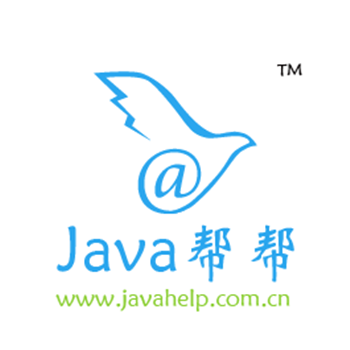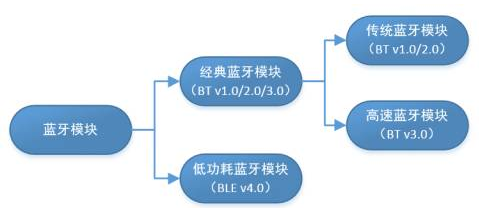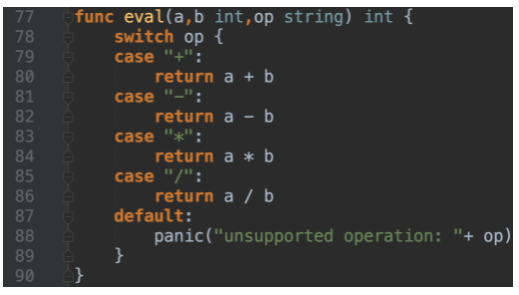Java基础-02总结关键字，标识符，注释，常量进制，变量数据类型

+关注继续查看

1:关键字(掌握)

(1)被Java语言赋予特定含义的单词
(2)特点：

(3)注意事项：
A:goto和const作为保留字存在。

/*

A:goto和const是保留字
*/
class KeyWordDemo {
public static void main(String[] args) {
System.out.println("HelloWorld");
}
}

2:标识符(掌握)
(1)就是给类，接口，方法，变量等起名字的字符序列
(2)组成规则：
A:英文大小写字母
B:数字
C:$和_ (3)注意事项： A:不能以数字开头 B:不能是java中的关键字 C:区分大小写 (4)常见的命名规则(见名知意) A:包 全部小写 单级包：小写 举例：liuyi,com 多级包：小写，并用.隔开 举例：cn.itcast,com.baidu B:类或者接口 一个单词：首字母大写 举例：Student,Demo 多个单词：每个单词首字母大写 举例：HelloWorld,StudentName C:方法或者变量 一个单词：首字母小写 举例：name,main 多个单词：从第二个单词开始，每个单词首字母大写 举例：studentAge,showAllNames() D:常量 全部大写 一个单词：大写 举例：PI 多个单词：大写，并用_隔开 举例：STUDENT_MAX_AGE /* 标识符：就是给类，接口，方法，变量等起名字。 组成规则： A:英文字母大小写 B:数字字符 C:$和_

A:不能以数字开头
B:不能是Java中的关键字
C:Java语言严格区分大小写

class Student {}
class S{}

cn
itcast

*/
class MakeNameDemo {
public static void main(String[] args) {
//正确做法
int x = 100;

//不能以数字开头
//int 1y = 100;
int y1 = 100;

//不能是Java中的关键字
//int public = 100;
int Public = 100;
}
}

3:注释(掌握)
(1)就是对程序进行解释说明的文字
(2)分类：
A:单行注释 //
B:多行注释 /**/
C:文档注释(后面讲) /** */

/*

Java中注释的分类及格式

*/

//这是我的注释案例
class ZhuShiDemo {
//main方法是主方法
//是程序的入口
//被jvm调用
public static void main(String[] args) {
System.out.println("好好学习,天天向上");
}
}

(3)把HelloWorld案例写了一个带注释的版本。

/*

A:要写一个Java程序，必须定义类
B:把数据能够输出，说明我们的程序是可以独立运行的，而程序要独立运行，必须定义main方法
C:把数据输出在控制台，必须使用输出语句

A:java语言提供了一个关键字：class用来定义类，后面跟的是类名
B:main方法的格式是固定的：
public static void main(String[] args) {

}
C:输出语句的格式是固定的：
System.out.println("HelloWorld");
"HelloWorld"这个内容是可以改变的
*/

//这是我的HelloWorld案例
class HelloWorld {
/*

main方法是程序的入口

*/
public static void main(String[] args) {
//为了把数据显示在控制台，我们就使用了输出语句
System.out.println("HelloWorld");
}
}

(4)注释的作用
A:解释说明程序，提高了代码的阅读性。
B:可以帮助我们调试程序。

/*

A:解释说明程序，提高程序的阅读性
B:可以帮助我们调试程序。

*/
class ZhuShiDemo2 {
public static void main(String[] args) {
System.out.println("林青霞");
System.out.println("王祖贤");
System.out.println("刘亦菲");
System.out.println("范冰冰");
}
}

4:常量(掌握)
(1)在程序执行的过程中，其值不发生改变的量
(2)分类：
A:字面值常量
B:自定义常量(后面讲)
(3)字面值常量
A:字符串常量 "hello"
B:整数常量 12,23
C:小数常量 12.345
D:字符常量 'a','A','0'
E:布尔常量 true,false
F:空常量 null(后面讲)
(4)在Java中针对整数常量提供了四种表现形式
A:二进制 由0，1组成。以0b开头。
B:八进制 由0，1，...7组成。以0开头。
C:十进制 由0，1，...9组成。整数默认是十进制。
D:十六进制 由0，1，...9,a,b,c,d,e,f(大小写均可)组成。以0x开头。

/*

A:字面值常量
B:自定义常量(后面讲)

A:字符串常量 用双引号括起来的内容。

B:整数常量 所有的整数

C:小数常量 所有的小数

D:字符常量 用单引号括起来的内容

E:布尔常量 比较特殊

F:空常量 后面讲

*/
class ConstantDemo {
public static void main(String[] args) {
//字符串常量的输出
System.out.println("hello");

//整数常量的输出
System.out.println(100);

//小数常量的输出
System.out.println(100.10);

//字符常量的输出
System.out.println('a');
System.out.println('A');
System.out.println('0');
//这个是有问题的
//System.out.println('ab');

//布尔常量的输出
System.out.println(true);
System.out.println(false);
}
}

5:进制转换(了解)
(1)其他进制到十进制

(2)十进制到其他进制

(3)进制转换的快速转换法
A:十进制和二进制间的转换
8421码。
B:二进制到八进制，十六进制的转换

/*

*/
class JinZhiDemo {
public static void main(String[] args) {
System.out.println(100); //十进制

System.out.println(0b100); //二进制
System.out.println(0100); //八进制
System.out.println(0x100); //十六进制
}
}

1：得到下面数据的十进制值：
0b10101
=1*2^4 + 1*2^2 + 1*2^0
=16 + 4 + 1
=21

0123
=1*8^2 + 2*8^1 + 3*8^0
=64 + 16 + 3
=83
0x3c
=3*16^1 + c*16^0
=48 + 12
=60

2：得到下面数据的二进制，十进制，十六进制
52分别得到二进制，十进制，十六进制

52 / 2 = 26 0
26 / 2 = 13 0
13 / 2 = 6      1
6  / 2 = 3      0
3  / 2 = 1      1
1  / 2 = 0      1

0b110100

52 / 8 = 6 4
6  / 8 = 0      6

064

52 / 16 = 3 4
3  / 16 = 0 3

0x34

3:有符号数据表示法的练习
A:已知某数X的原码为10110100B，试求X的补码和反码。

B:已知某数X的补码11101110B，试求其原码。

6:变量(掌握)
(1)在程序的执行过程中，其值在某个范围内可以发生改变的量
(2)变量的定义格式：
A:数据类型 变量名 = 初始化值;
B:数据类型 变量名;
变量名 = 初始化值;

/*

A:作用域

B:初始化值

a:数据类型 变量名 = 初始化值;
b:数据类型 变量名;
变量名 = 初始化值;

C:在一行上建议只定义一个变量

*/
class DataTypeDemo2 {
public static void main(String[] args) {
//定义变量
int x = 100;

//错误，不能有同名的
//int x = 200;

//定义变量必须给值
//int y;
//System.out.println(y);

int z;
z = 100;
System.out.println(z);

//在一行上定义多个变量
//int a = 10; int b = 20; int c  = 30;
//上面的写法可以，但是不建议。
int a = 10;
int b = 20;
int c  = 30;

//int d, e;
//d = 40;
//e = 50;

//int f,int g; //错误
//int h; int i; //正确
}
}

7:数据类型(掌握)
(1)Java是一种强类型语言，针对每种数据都提供了对应的数据类型。
(2)分类：
A:基本数据类型：4类8种
B:引用数据类型：类，接口，数组。
(3)基本数据类型
A:整数 占用字节数
byte 1
short 2
int 4
long 8
B:浮点数
float 4
double 8
C:字符
char 2
D:布尔
boolean 1

/*

A:基本数据类型
B:引用数据类型(类,接口,数值)

A:整数 占用字节数
byte 1
short 2
int 4
long 8

B:浮点数
float 4
double 8

C:字符
char 2
D:布尔
boolean 1

*/
class DataTypeDemo {
public static void main(String[] args) {
//定义变量的格式：
//数据类型 变量名 = 初始化值;

//定义一个字节变量
byte b = 10;
System.out.println(10);
System.out.println(b);

//定义一个短整型变量
short s = 100;
System.out.println(s);

//定义一个整型变量
int i = 1000;
System.out.println(i);

//超过了int的范围
//int j = 1000000000000;
long j = 1000000000000L;
//long j = 100L;
System.out.println(j);

//定义浮点数据变量
float f = 12.345F;
System.out.println(f);

double d = 12.345;
System.out.println(d);

//定义字符变量
char ch = 'a';
System.out.println(ch);

//定义布尔变量
boolean flag = true;
System.out.println(flag);
}
}

8:数据类型转换(掌握)
(1)boolean类型不参与转换
(2)默认转换
A:从小到大
B:byte,short,char -- int -- long -- float -- double
C:byte,short,char之间不相互转换，直接转成int类型参与运算。

/*
+是一个运算符(我们等会讲解)。做加法运算的。

boolean类型不能转换为其他的数据类型

A:byte,short,char—int—long—float—double
B:byte,short,char相互之间不转换，他们参与运算首先转换为int类型
*/
class DataTypeDemo3 {
public static void main(String[] args) {
//直接输出的方式做加法
//System.out.println(3 + 4);

//两个int类型做加法
int x = 3;
int y = 4;
int z = x + y;
System.out.println(z);

//定义一个byte类型，一个int类型，做加法
byte a = 3;
int b = 4;
System.out.println(a + b);

//可能损失精度
//byte c =  a + b;
int c = a + b;
System.out.println(c);
}
}

(3)强制转换
A:从大到小
B:可能会有精度的损失，一般不建议这样使用。
C:格式：

/*

*/
class DataTypeDemo4 {
public static void main(String[] args) {
byte a = 3;
int b = 4;

//这个肯定没有问题
//int c = a + b;

//byte c = 7;
//这个是有问题的
//byte c = a + b;
//用强制类型转换改进
byte c = (byte) (a + b);
System.out.println(c);
}
}

(4)思考题和面试题：
A:下面两种方式有区别吗?
float f1 = 12.345f;
float f2 = (float)12.345;

/*

double d = 12.345;
float f = d;

float f1 = (float)12.345;
float f2 = 12.345f;

f1其实是通过一个double类型转换过来的。

*/
class DataTypeDemo5 {
public static void main(String[] args) {
//把double赋值给float，加了强制类型转换
double d = 12.345;
float f = (float)d;

//看看下面两个定义有没有区别呢?
float f1 = (float)12.345;
float f2 = 12.345F;
}
}

B:下面的程序有问题吗，如果有，在哪里呢?
byte b1 = 3;
byte b2 = 4;
byte b3 = b1 + b2;
byte b4 = 3 + 4;

/*

byte b1=3,b2=4,b;
b=b1+b2;
b=3+4;

b = b1 + b2;是有问题的。

*/
class DataTypeDemo6 {
public static void main(String[] args) {
//定义了三个byte类型的变量，b1，b2，b3
//b1的值是3，b2的值是4，b没有值
byte b1 = 3,b2 = 4,b;

//b = b1 + b2; //这个是类型提升，所有有问题

b = 3 + 4; //常量，先把结果计算出来，然后看是否在byte的范围内，如果在就不报错。
}
}

C:下面的操作结果是什么呢?
byte b = (byte)130;

/*
byte b = 130;有没有问题?如果我想让赋值正确，可以怎么做?结果是多少呢?

*/
class DataTypeDemo7 {
public static void main(String[] args) {
//因为byte的范围是：-128到127。
//而130不在此范围内，所以报错。
//byte b = 130;

//我们可以使用强制类型转换
byte b = (byte) 130;

//结果是多少呢?
System.out.println(b);
}
}
/*

A:获取130这个数据的二进制。
00000000 00000000 00000000 10000010

B:做截取操作，截成byte类型的了。
10000010

C:已知补码求原码。

*/

D:字符参与运算

'a' 97
'A' 65
'0' 48

System.out.println('a');
System.out.println('a' + 1);

/*

'a' 97
'A' 65
'0' 48
*/
class DataTypeDemo8 {
public static void main(String[] args) {
//直接输出一个字符
System.out.println('a'); //a
//输出一个字符和一个整数做加法
System.out.println('a'+1); //98
}
}

E:字符串参与运算

System.out.println("hello"+'a'+1);
System.out.println('a'+1+"hello");
System.out.println("5+5="+5+5);
System.out.println(5+5+"=5+5");

/*

*/
class DataTypeDemo9 {
public static void main(String[] args) {
System.out.println("hello"+'a'+1); //helloa1
System.out.println('a'+1+"hello"); //98hello

System.out.println("5+5="+5+5); //5+5=55
System.out.println(5+5+"=5+5"); //10=5+5
}
}

(1):在定义Long或者Float类型变量的时候，要加L或者f。

byte，short在定义的时候，他们接收的其实是一个int类型的值。

(2):byte值的问题
byte b1 = 127;
byte b2 = (byte)128; //-128
byte b3 = (byte)129; //-127
byte b4 = (byte)130; //-126

byte的范围：-128 ~ 127

128：10000000
-128：10000000 (这里的1即是符号位，也是数值位)

(3):数据类型转换之默认转换
byte,short,char -- int -- long -- float -- double

long: 8个字节
float：4个字节

A:它们底层的存储结构不同。
B:float表示的数据范围比long的范围要大
long：2^63-1
float：3.4*10^38 > 2*10^38 > 2*8^38 = 2*2^3^38 = 2*2^114 > 2^63-1

( 4):Java语言中的字符char可以存储一个中文汉字吗?为什么呢?

Java语言采用的是Unicode编码。Java基础-18总结Map,HashMap,HashMap与Hashtable区别,Collections工具类

2452 0BLE协议学习总结

1954 0593 0Python零基础学习笔记（四）——数据类型
python数据类型：Number（数字）：包括整数/浮点数（小数）/复数String（字符串）Boolean（布尔值）None（空值）list（列表）tuple（元组）dict（字典）set（集合）
1235 0Go实战(二)-变量、语句、函数、指针、关键字（下）
Go实战(二)-变量、语句、函数、指针、关键字
10 0Java基础-02总结关键字，标识符，注释，常量进制，变量数据类型

1352 0Java基础-23总结多线程,线程实现Runnable接口,线程名字获取和设置,线程控制,线程安全,同步线程

2784 0OCCI处理CHAR类型字符串变量的不同
675 0Oracle连接不上：ORA-12154:TNS无法解析指定的连接标识符
2785 0JavaScript基础（二）变量

1026 0

Java帮帮，全国领先的IT新媒体，从创立以来一直坚持不懈，把简单学习，快乐学习，高效学习的经营理念，发扬光大；让每一位想要学习，想要凭借自己力量改变未来的人，都能轻松的获取学习资源，从而实现自己的人生价值。
44

0

《2021云上架构与运维峰会演讲合集》

《零基础CSS入门教程》

《零基础HTML入门教程》